NEET  >  Test: Addition & Subtraction of Vectors - Graphical Method (NCERT)

# Test: Addition & Subtraction of Vectors - Graphical Method (NCERT) - NEET

Test Description

## 5 Questions MCQ Test Physics Class 11 - Test: Addition & Subtraction of Vectors - Graphical Method (NCERT)

Test: Addition & Subtraction of Vectors - Graphical Method (NCERT) for NEET 2023 is part of Physics Class 11 preparation. The Test: Addition & Subtraction of Vectors - Graphical Method (NCERT) questions and answers have been prepared according to the NEET exam syllabus.The Test: Addition & Subtraction of Vectors - Graphical Method (NCERT) MCQs are made for NEET 2023 Exam. Find important definitions, questions, notes, meanings, examples, exercises, MCQs and online tests for Test: Addition & Subtraction of Vectors - Graphical Method (NCERT) below.
Solutions of Test: Addition & Subtraction of Vectors - Graphical Method (NCERT) questions in English are available as part of our Physics Class 11 for NEET & Test: Addition & Subtraction of Vectors - Graphical Method (NCERT) solutions in Hindi for Physics Class 11 course. Download more important topics, notes, lectures and mock test series for NEET Exam by signing up for free. Attempt Test: Addition & Subtraction of Vectors - Graphical Method (NCERT) | 5 questions in 5 minutes | Mock test for NEET preparation | Free important questions MCQ to study Physics Class 11 for NEET Exam | Download free PDF with solutions
 1 Crore+ students have signed up on EduRev. Have you?
Test: Addition & Subtraction of Vectors - Graphical Method (NCERT) - Question 1

### Which of the following is not a property of a null vector?

Detailed Solution for Test: Addition & Subtraction of Vectors - Graphical Method (NCERT) - Question 1

Multiplying any vector by zero will result in a null vector.
So, the property of null vector is wrongly mention in option (C)
Hence, the correct option is (C).

Test: Addition & Subtraction of Vectors - Graphical Method (NCERT) - Question 2

### Given  a + b + c + d = 0, which of the following statements are correct.

Detailed Solution for Test: Addition & Subtraction of Vectors - Graphical Method (NCERT) - Question 2

(a) Incorrect, because a + b + c + d can zero in many ways other than that (vector a, b, c, and d) must each be a null vector.
(b) Correct, as (vector a + b + c + d = 0;) (vector a + c) = -(vector b + d).
Thus, (vector a + b) is equal to negative of( vector b + d) and hence the statement that magnitude of (vector A + C) is equal to the magnitude of (vector b + d) is correct.
(c) correct, Since (vector a + b + c + d = 0'
(vector a = -(b + c + d))
Thus, magnitude of vector a is equal to (vector b + c + d). The sum of the magnitude of (vectors b + c) and d may be greater than or equal to that of vector a. Hence the statement that the magnitude of vector a can never be greater than the sum of the magnitude of (vector b, c and d) is correct.
(d) Correct, because (vector a + b + c + d = 0;) hence ((vector b + c) + a + d = 0)
The resultant sum of three (vectors b + c, + a + d) can be zero only if (vector b + c) is in the plane of (vector a and d). In case vector a and d are collinear, (vector b + c) must be the line of (vector a and d.) Hence the given statement is correct.

Test: Addition & Subtraction of Vectors - Graphical Method (NCERT) - Question 3

### Two vectors A and B inclined at an angle θ have a resultant R which makes an angle α with A. If the directions of A and B are interchanged, the resultant will have the same

Detailed Solution for Test: Addition & Subtraction of Vectors - Graphical Method (NCERT) - Question 3

Neither the magnitude of vectors nor the angle between the vectors is changed. So, magnitude of the resultant remains unchanged. However, the direction of the resultant will be changed.

Test: Addition & Subtraction of Vectors - Graphical Method (NCERT) - Question 4

Three forces of magnitude 6 N, 6 N and √72 N  act as a corner of a cube along three sides as shown in fig. Resultant of these forces is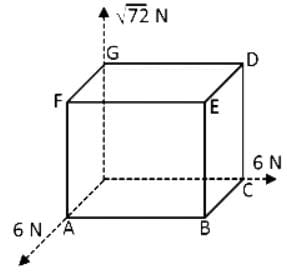Detailed Solution for Test: Addition & Subtraction of Vectors - Graphical Method (NCERT) - Question 4

Let sides OC, OG and OA represent x, y and z respectively.

Resultant of forces = 6i + 72 j + 6j = vector R

R = √(36 + 72 + 36) = 144 = 12 N

Resultant of OA and OC is along OB and of magnitude 72

(∵ they are perpendicular)

∴ Resultant of OC, OG and OA is same as OB 72 + OG √72

Its resultant will be along OE.

Test: Addition & Subtraction of Vectors - Graphical Method (NCERT) - Question 5

Rain is falling vertically with a speed of 35ms−1. Winds starts blowing after sometime with a speed of 12ms−1 in east to west direction. At what angle with the vertical should a boy waiting at a bus stop hold his umbrella to protect himself from rain?

Detailed Solution for Test: Addition & Subtraction of Vectors - Graphical Method (NCERT) - Question 5

The velocity of the rain and the wind are represented by the vectors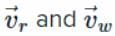as shown in the figure. To protect himself from the rain the boy should hold his umbrella in the direction of resultant velocity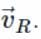If θ is the angle which resultant velocity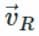makes with the vertical, then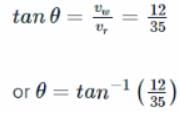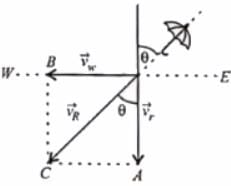## Physics Class 11

130 videos|483 docs|210 tests
Information about Test: Addition & Subtraction of Vectors - Graphical Method (NCERT) Page
In this test you can find the Exam questions for Test: Addition & Subtraction of Vectors - Graphical Method (NCERT) solved & explained in the simplest way possible. Besides giving Questions and answers for Test: Addition & Subtraction of Vectors - Graphical Method (NCERT), EduRev gives you an ample number of Online tests for practice

## Physics Class 11

130 videos|483 docs|210 tests

### How to Prepare for NEET

Read our guide to prepare for NEET which is created by Toppers & the best Teachers(Scan QR code)